# Math Brain Teasers 13:: Can You Solve this Math Riddle

in STEMGeeks2 years ago (edited)

Hey All;

It's been a while that I posted a Math Brain Teaser; was a bit bussy with other stuff. So without a further do let's get directly looking at our Math Brain Teasers 13; solve this Math Riddle. Check the given equation in the image below and create a new equation using the numbers in the image and mathematical symbol. I hope this isn't going to be too hard, all I can say is that it's not that complex a simple equation that everyone can solve...its that easy.. All you need to do is apply simple logic to get to the answer.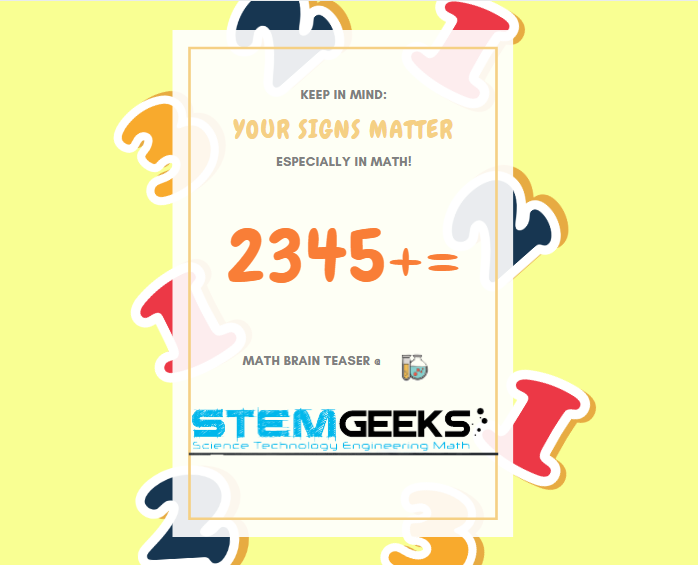Hint:: Is this maths puzzle that complicated to solve and do we require a Hint? I don't think so that a hint would be required to solve this equation. In case if you still require a hint, please let me know in the comment box below and I'll pass on something that should help you come up with the new equation to solve this maths puzzle.

##### Math Brain Teasers 12:: Solve the Maths Grid Puzzle? - Solved with Explanation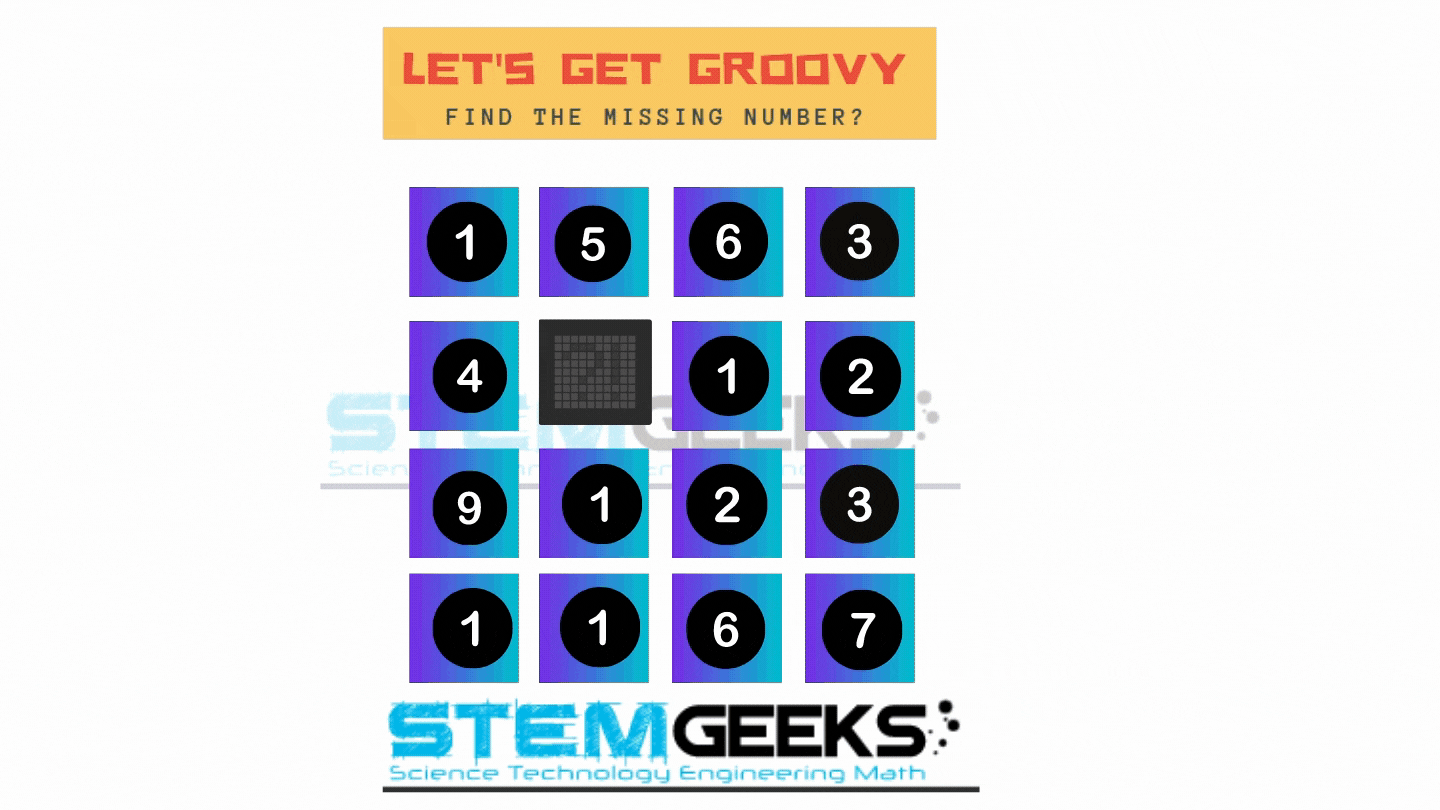• Step 1. Add up all the Rows, and the answer that would come is 15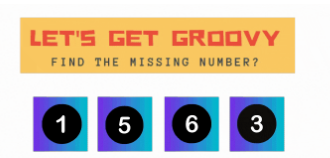Lets take the first row as an example; adding::

### 1 + 5 + 6+ 3 = 15

• Step 2. Add all columns; also the answer that would come is 15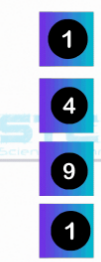Now; Lets take the first Column; as an example; adding::

### 1 + 4 + 9 + 1 = 15

In the final step lets have a look at the row for which we need to find the number that replaces the question mark::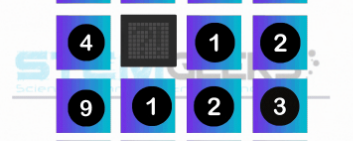### 4 + 8 + 1 + 2 = 15 [The question mark is replaced by the letter 8 ]

Hence the correct answer to our **Math Brain Teasers 12 :: Solve the Maths Grid Puzzle? = 8

### STEM token GiveAway

I'll be again doing a giveaway of STEM tokens to the lucky random winner with the correct answer. For the last contest, which was Patterns Within the Triangle?.

We had 6 entries & all of them were correct. I'm going to use the comment picker tool to randomly select the WINNER..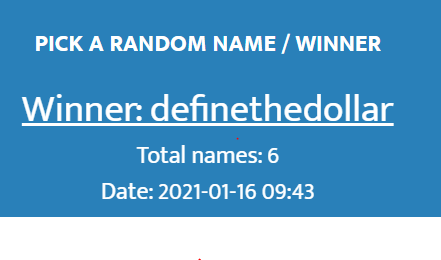Congratulations @definethedollar; You WIN yourself 10 STEM tokens. You should be having the rewards in your STEM Wallet Soon.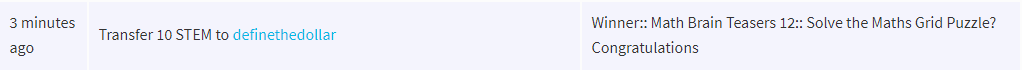### Math Quote for the Day::

Here is the motivation to solve this Maths Grid Puzzle; find out what should replace the question mark..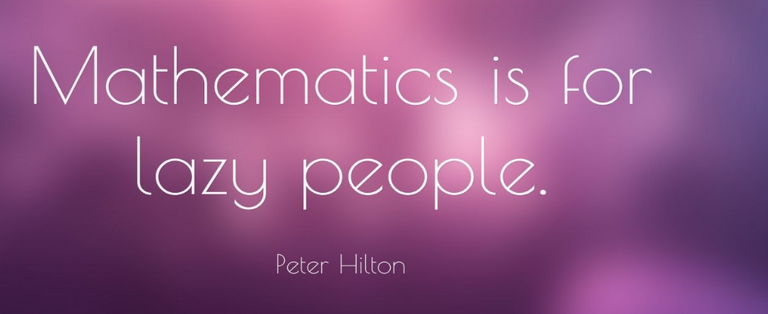source::

If you like my work, then please spread the Word.. that we do have the Math Brain Teasers competition here @StemGeeks platform. Reblog is much appreciated.

Best RegardsFind Me on the Other Social Media Platforms::PS:: All the Maths Brain Teasers; are made by me using the Pro Canva License Version

Sort:

4 + 5 = 3^2 (9)

Better than the desk question mate lol ;-)

PS IN your post, you didn't say if you use the numbers once only!

If we split the "+" in two lines:

5-4=3-2
1=1

4+3=5+2